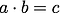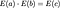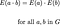# What are zk-SNARKs? — A Review of Homomorphisms

I am studying homomorphic hidings using this post from the Zcash blog and this article from Wikipedia as a reference. I am writing about homomorphisms and homomorphic hidings as a way to synthesize what I’ve learned.

# Homomorphisms

A homomorphism is a map between two algebraic structures that preserves the operations of both structures.

Let’s say we have groups G and H. We can define a map E that takes elements of G and sends them to elements of H:

The map E is a homomorphism if the arithmetic done in G still works in H after applying the map. So if we can do this multiplication in G:a, b, and c are elements of G and the ‘dot’ is the operation used in G

Then this should still make sense in H:E(a), E(b), and E(c) are elements of H and the dot is the operation used in H

If this is true for all the elements of G, then E is a homomorphism. A more succinct way of stating this property is:This is the standard way of showing a map is a homomorphism.

An example of a homomorphism could be a map from the even integers under addition to all integers under addition. The map would associate 0 with 0, 2 with 1, 4 with 2, 6 with 3, and so on in both directions. Each even integer would be matched with the integer that is half as large. In the “even integers” group: 8 + 14 = 22. This corresponds to the equation 4 + 7 = 11 in the “all integers” group. It is not hard to show that this is true for all equations like these for this homomorphism.

Homomorphisms don’t seem to be very good at encrypting anything, usually. Since the homomorphism respects arithmetic, it can’t “scramble” the elements very much. A simple homomorphism like the one I just described above is very easy to reverse. If you give me an integer I can easily find its preimage by multiplying it by 2.

There are some homomorphisms that are not easy to reverse. That is, if you know that E(some input) = 47, it is extremely difficult to figure out what the original input was. Such a homomorphism could be used for encryption.

# Homomorphic Encryption

The RSA cryptosystem is based on a simple homomorphism. Called Unpadded RSA, the homomorphism is defined like this:

This is a homomorphism because:

The second-to-last line shown above is due to the fact that the mod operation respects multiplication. (That multiplication is done in the group serving as the codomain, so is also done mod m).

This homomorphism has some of the properties of a hiding: Even if you know m and e, it is usually difficult to find a from E(a) as long as e and m are chosen carefully. This is known as the “RSA Problem” and the (assumed) difficulty of this problem is what gives the RSA cryptosystem its power. Unfortunately this homomorphism lacks a critical property that could make it a hiding. See the problem in my next post.

Written by